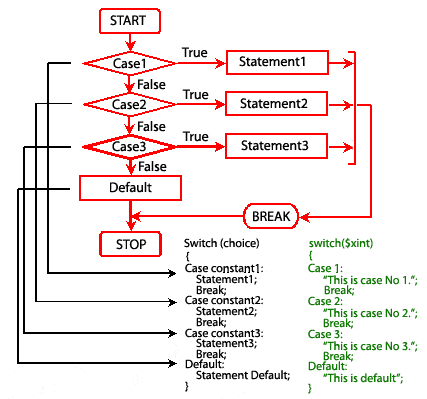# PHP Switch statement

## Description

The control statement which allows us to make a decision from the number of choices is called a switch-case-default. It is almost similar to a series of if statements on the same expression.

Syntax:

```switch (expression )
{
case constant1:
execute the statement;
break;
case constant2:
execute the statement;
break;
case constant3:
execute the statement;
break;
.........
default:
execute the statement;
}
```

The expression following the keyword switch can be a variable or any other expression like an integer, a string, or a character. Each constant in  each case must be different from all others.

When we run a program containing the switch statement at first the expression following the keyword switch is evaluated. The value it gives is then matched one by one against the constant values that follow the case statements. When a match is found the program executes the statements following that case. If no match is found with any of the case statements, only the statements following the default are executed.

Example:

In the following example \$xint is equal to 3, therefore switch statement executes the third echo statement.

``````<?php
\$xint=3;
switch(\$xint) {
case 1:
echo "This is case No 1.";
break;
case 2:
echo "This is case No 2.";
break;
case 3:
echo "This is case No 3.";
break;
case 4:
echo "This is case No 4.";
break;
default:
echo "This is default.";
}
?>``````

Output:

`This is case No 3.`

Pictorial presentation of switch loopPrevious: continue statement
Next: declare statement

﻿

## PHP: Tips of the Day

Members of objects or classes can be accessed using the object operator (->) and the class operator (::).

Example:

```class MyClass {
public \$a = 1;
public static \$b = 2;
const C = 3;
public function d() { return 4; }
public static function e() { return 5; }
}
\$object = new MyClass();
var_dump(\$object->a); // int(1)
var_dump(\$object::\$b); // int(2)
var_dump(\$object::C); // int(3)
var_dump(MyClass::\$b); // int(2)
var_dump(MyClass::C); // int(3)
var_dump(\$object->d()); // int(4)
var_dump(\$object::d()); // int(4)
var_dump(MyClass::e()); // int(5)
\$classname = "MyClass";
var_dump(\$classname::e()); // also works! int(5)
```

Note that after the object operator, the \$ should not be written (\$object->a instead of \$object->\$a). For the class operator, this is not the case and the \$ is necessary. For a constant defined in the class, the \$ is never used.

Also note that var_dump(MyClass::d()); is only allowed if the function d() does not reference the object:

```class MyClass {
private \$a = 1;
public function d() {
return \$this->a;
}
}
\$object = new MyClass();
var_dump(MyClass::d()); // Error!
```

This causes a 'PHP Fatal error: Uncaught Error: Using \$this when not in object context'

These operators have left associativity, which can be used for 'chaining':

```class MyClass {
private \$a = 1;

\$this->a += \$a;
return \$this;
}

public function get() {
return \$this->a;
}
}
\$object = new MyClass();
```

These operators have the highest precedence (they are not even mentioned in the manual), even higher that clone. Thus:

```class MyClass {
private \$a = 0;
\$this->a += \$a;
return \$this;
}
public function get() {
return \$this->a;
}
}
\$o1 = new MyClass();
var_dump(\$o1->get()); // int(2)
var_dump(\$o2->get()); // int(2)
```

The value of \$o1 is added to before the object is cloned!

Note that using parentheses to influence precedence did not work in PHP version 5 and older (it does in PHP 7):

```// using the class MyClass from the previous code
\$o1 = new MyClass();
\$o2 = (clone \$o1)->add(2); // Error in PHP 5 and before, fine in PHP 7
var_dump(\$o1->get()); // int(0) in PHP 7
var_dump(\$o2->get()); // int(2) in PHP 7
```